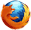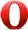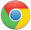# JavaScript round() 方法JavaScript Math 对象

## 实例

round() 方法可把一个数字舍入为最接近的整数：

Math.round(2.5);

3

## 定义和用法

round() 方法可把一个数字舍入为最接近的整数。

## 浏览器支持Math.round(x)

x 必需。必须是数字。

Number 最接近的整数。

## 技术细节

 JavaScript 版本： 1

## 实例

var a=Math.round(2.60);
var b=Math.round(2.50);
var c=Math.round(2.49);
var d=Math.round(-2.60);
var e=Math.round(-2.50);
var f=Math.round(-2.49);

a,b,c,d,e, 和 f 输出结果：

3
3
2
-3
-2
-2JavaScript Math 对象